# Sweep 2.0geometry node

Creates a surface by sweeping a cross section curve along a spine curve.

## Overview

This node takes a cross-section curve from the second input, distributes copies of it along a spine curve from the first input, and creates a surface between the distributed cross-section curves. It is an extremely versatile workhorse for generating procedural geometry.This node will accept polylines, NURBS curves, or Beziér curves. By default the type of input curves determine the type of surface (for example, polylines produce polygons, NURBS curves produce NURBS surfaces), however you can override the surface type using the Primitive type parameter on the Construction tab.

Instead of using modeled cross-sections connected to the second input, you can use automatic circle, square, or line cross-sections using the Surface Shape parameter.

This node can handle multiple spines, and/or multiple cross-sections.

• If there are multiple curves in the first (spine) input, the cross-section(s) is/are swepat along each spine curve separately.

• If there are multiple curves in the second (cross-section) input, the cross-sections are ditributed along the curve length according to the Cross section order parameter on the Construction tab.

This node can handle closed spines and/or closed cross-sections. (Closed means that the curve is a loop — the first and last point of the curve are the same.)

• If both the spine and cross-section are open, the node creates an open surface.

• If either the spine or cross-section are closed, the node creates a tube.

When one curve is open and the other closed, creating a tube, you can automatically add end-cap geometry using the End cap type parameter on the Surface tab.

• If both the spine and cross-section are closed, the node creates a torus.

## Positioning the curves

• Spine curves can be anywhere in space.

• This node assumes all cross section curves are modeled at the origin in the XY plane, with +Y up direction.

Do not model the cross-section curve on (or at the beginning of) the spine curve.

• When the curve is flat (planar), the node assumes it should orient the up direction of the cross-sections perpendicular to the curve plane.

After construction, you can adjust the orientation of the cross-section curve(s) relative to the spine using the Roll and Yaw parameters.

## Inputs

First (Backbone curves)

Should contain one or more spine curves. If the input has multiple curves, the cross-section(s) is/are surfaced along each spine separately.

Second (Cross section(s))

Should contain one or more cross-section curves. If the input has multiple curves,

Connecting modeled cross-section curves to the second input is optional. Instead, you can use automatic circle, square, or line cross-sections using the Surface Shape parameter.

## Parameters

Backbone Curve Group

If this is blank, the node uses all curves from the first input, or you can specify a group or set of curves using group syntax. You can also click theReselect button next to this field to select the curves in the viewport.

Cross Section Group

If this is blank, the node uses all curves from the second input, or you can specify a group or set of curves using group syntax. You can also click theReselect button next to this field to select the curves in the viewport.

## Surface

Surface Shape

What to use as the cross-section shape. The default is to use curves connected to this node’s second input.

Second Input Cross Sections

Use the curve or curves from the second input (taking into account the Cross section group field) as the cross-section(s).

Round Tube

Use a circle as the cross-section, producing a round tube. Scale the tube with the Radius parameter and set the number of sides the circle has with the Columns parameter.

Square Tube

Use a square as the cross-section, producing a boxy tube. Scale the tube with the Width parameter and set the number of subdivisions along each side with the Columns parameter.

Ribbon

Use a line as the cross-section, producing a ribbon shape. Scale the ribbon with the Width parameter and set the number of subdivisions across the line with the Columns parameter.

Surface Type

The surface topology of the generated surface.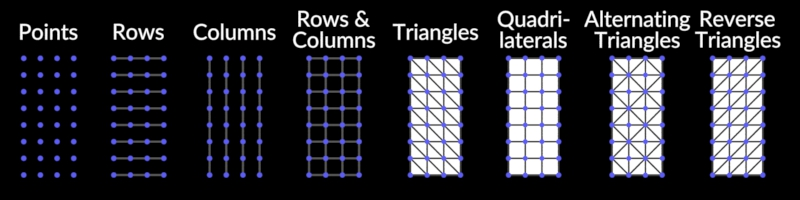The Columns option is useful for creating many curves from each input curve.

Points

Don’t create any primitives, only points.

Rows

Create a curve for each cross section in the grid.

Columns

Create a curve for each vertex around the grid, spanning the full curve.

Rows and Columns

Create curves corresponding with both the Rows option and the Columns option.

Triangles

Create triangles splitting the grid quads in a single direction.

Create the grid quads as is.

Alternating Triangles

Create triangles splitting the grid quads in alternating directions.

Reverse Triangles

Create triangles splitting the grid quads in a single direction opposite that of the Triangles option.

Scale Cross Sections

When Surface shape is "Second Input", this scales the size of all cross-sections.

Columns

When Surface shape is "Round tube", "Square tube", or "Ribbon", this controls the density of the cross-section shape. For round, this is the number of sides the circle has. For square, this is the number of subdivisions along each side. For ribbon, this is the number of subdivisions across.

When Surface shape is "Round tube", the radius of the tube.

Width

When Surface shape is "Square tube" or "Ribbon", the width of the tube/ribbon.

Reverse Cross Sections

Turn this on to reverse the normals of the generated surface.

When this is on, the node treats cross section primitives as if they were reversed, which reverses normals on the generated surface.Stretch Around Turns

Stretch cross-sections in the direction of curvature around turns in the spine. (Default on.) This avoids the cross-section appearing squished in turns. This is usually what you want, unless you have set Surface type to "rows" or "columns".Max Stretch

When Stretch Around Turns is on, the maximum amount that cross sections will be stretched. The default is 10 (maximum 10× stretch). This prevents the cross-section from scaling wildly if, for example, the spine curve doubles back on itself.

End Caps

End Cap Type

When the spine curve is open and the cross-section curves are closed, the generated surface is a tube. The node can automatically create end-caps on the open ends of the tube.

None

Do not generate end-caps.

Single Polygon

Cap the ends with a single N-gon.

Grid

Cap the ends with a surface, controlled by the parameters below.

Side Single Polygon

When the spine is closed but the cross-sections are open, the sides of the generated surface are open rather then the ends. This closes the sides with an N-gon.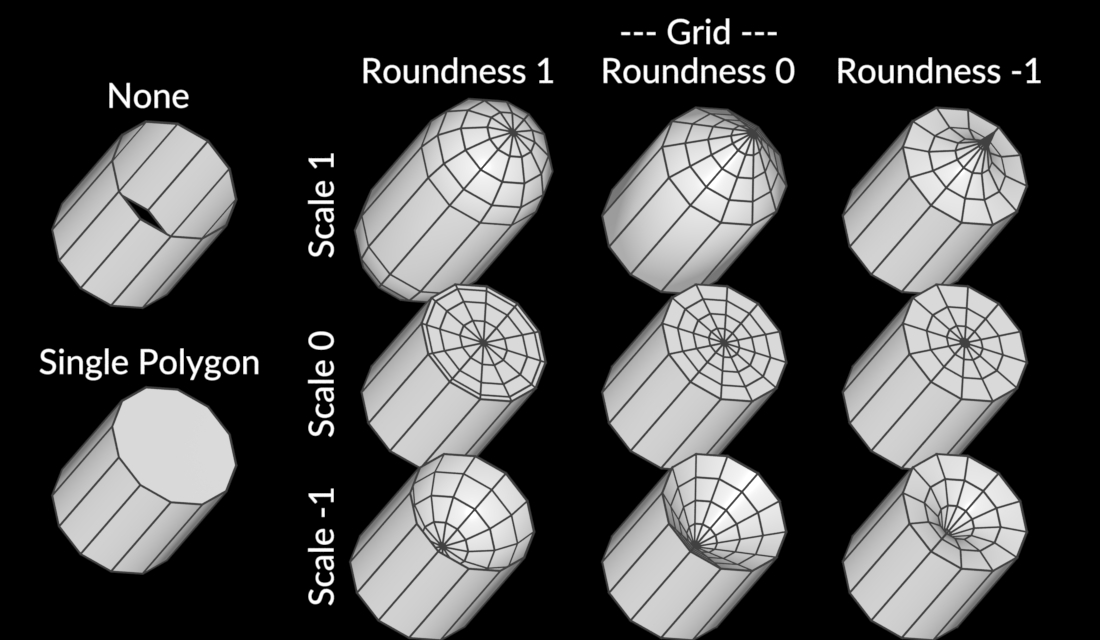Cap Divisions

When End Cap Type is Grid, the number of rows in the end-cap surfaces.

Triangular Poles

When End Cap Type is Grid and this is on, the poles of end caps are made with triangles. When this is off (the default), the poles are made with quads with duplicate vertices.

End Cap Scale

When End Cap Type is Grid, this is the amount each end-cap "bulges out" from each end of the tube. Set this to `0` for no bulge, or to a negative number for an inward bulge.

End Cap Roundness

When End Cap Type is Grid, and End cap scale is not `0`, this controls the curvature of the bulge. `1` is convex circular, `0` is linear, and `-1` is concave circular.End Caps Group

When this is on, the output geometry includes a group by this name, containing all end-cap geometry. This lets you treat the end-caps separately if necessary in down-stream nodes.

Scale

Apply Scale Along Curve

Scales the generated surface across the length of the spine curve according to the Scale ramp parameter.

Scale Ramp

When Apply scale along curve is on, this ramp lets you interactively scale the surface inward and outward along the length of the spine curve.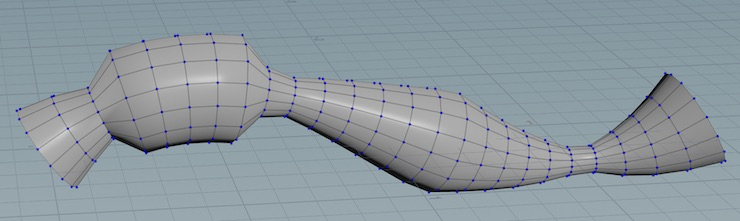## Rotation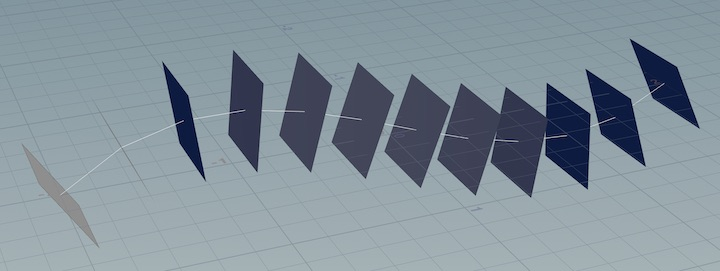Rotate Order

The order in which to apply the Pitch (around X/out), Yaw (around Y/up), and Roll or Twist (around Z/tangent) options below, when more than one can apply.

Pitch and Yaw may be useful to effectively apply a pre-transform to the cross sections before using them if they are not in the XY plane in the cross section input, which is why they default to being applied before the Roll or Twist.

These rotations are effectively applied to the cross sections before the transforms from the backbone curves, having the net effect of them being rotations in the space of the backbone curve frames.

Apply Roll or Twist

Apply the Roll and Twist parameters below to the cross-section curves.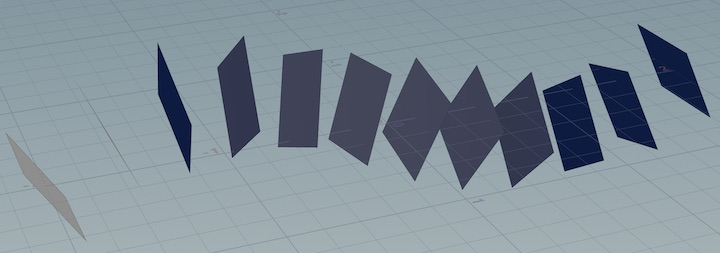Roll

A rotation, in degrees, around the spine curve direction, applied equally to every cross-section. You can use this to correct the orientation of the cross-section in relation to the curve.

Full Twists

A convenience to add full twists (for each interval set in the Twist per parameter). This node multiplies this by 360 and adds it to the number in Partial twist.

Partial Twist

The number of degrees of twist to add (for each interval set in the Twist per parameter).

Twist Per

The interval along each of which the total twist specified by the Full twists and Partial twist parameters is applied to the surface.

See the descriptions and image below.

Per Edge

The twist applies along each edge (the distance from each point in the curve to the next point).

Per Unit Distance

The twist applies each "world unit" of distance along the curve.

Scale by Attribute

The twist value is multiplied at each vertex by the value in the attribute named in the Twist ramp attribute paramter (this can be a vertex, point, primitive, or detail attribute). This product is applied as a roll at each vertex, not as an accumulating twist.

Per Full Curve by Edges

Applies the twist value once across the entire curve, divided equally among each edge.

Per Full Curve by Distance

Applies the twist value once across the entire curve, with the amount proportional to the distance along the curve.Twist Ramp Attribute

When Twist Per is "Scale By Attribute", this is the name of the vertex, point, primitive, or detail attribute to multiply by the total twist amount (full twists combined with partial twist). This product is applied as a roll at each vertex, not as an accumulating twist.

Apply Yaw

Apply the Yaw parameters below to the cross-section curves. Yaw is a rotation around the cross-section’s "up" vector, usually thought of as "turning left or right".Yaw

A rotation, in degrees, around the cross-section’s "up" vector, applied equally to every cross-section. (This is usually thought of as "turning left or right".) You can use this to correct the orientation of the cross-section relative to the curve.

Incremental Yaw

A rotation, in degrees, around the cross-section’s "up" vector (usually thought as "turning left or right"), to apply along each interval set in the Yaw per parameter.

Yaw Per

The interval along each of which the total rotation specified by the Incremental Yaw parameter is applied to the surface.

Per Edge

The yaw applies along each edge (the distance from each point in the curve to the next point).

Per Unit Distance

The yaw applies each "world unit" of distance along the curve.

Scale by Attribute

The yaw value is multiplied at each vertex by the value in the attribute named in the Yaw ramp attribute paramter (this can be a vertex, point, primitive, or detail attribute). This product is applied as a yaw at each vertex, not as an accumulating yaw.

Per Full Curve by Edges

Applies the yaw value once across the entire curve, divided equally among each edge.

Per Full Curve by Distance

Applies the yaw value once across the entire curve, with the amount proportional to the distance along the curve.

Yaw Ramp Attribute

When Yaw Per is "Scale By Attribute", this is the name of the vertex, point, primitive, or detail attribute to multiply by Incremental Yaw. This product is applied as a yaw at each vertex, not as an accumulating yaw.

Apply Pitch

Apply the Pitch parameters below to the cross-section curves. Pitch is a rotation around the cross-section’s "out" vector, usually thought of as "tilting up or down".Pitch

A rotation, in degrees, around the cross-section’s "out" vector, applied equally to every cross-section. (This is usually thought of as "tilting up or down".) You can use this to correct the orientation of the cross-section relative to the curve.

Incremental Pitch

A rotation, in degrees, around the cross-section’s "out" vector (usually thought as "tilting up or down"), to apply along each interval set in the Yaw per parameter.

Pitch Per

The interval along each of which the total rotation specified by the Incremental Pitch parameter is applied to the surface.

Per Edge

The pitch applies along each edge (the distance from each point in the curve to the next point).

Per Unit Distance

The pitch applies each "world unit" of distance along the curve.

Scale by Attribute

The pitch value is multiplied at each vertex by the value in the attribute named in the Yaw ramp attribute paramter (this can be a vertex, point, primitive, or detail attribute). This product is applied as a pitch at each vertex, not as an accumulating pitch.

Per Full Curve by Edges

Applies the pitch value once across the entire curve, divided equally among each edge.

Per Full Curve by Distance

Applies the pitch value once across the entire curve, with the amount proportional to the distance along the curve.

Pitch Ramp Attribute

When Pitch Per is Scale By Attribute, this is the name of the vertex, point, primitive, or detail attribute to multiply by Incremental Pitch. This product is applied as a pitch at each vertex, not as an accumulating pitch.

## Construction

Cross Sections

Cross Section Order

How the node decides what cross section(s) to use a each point along the spine curve.

All Cross Sections at Each Curve Vertex

Within a single curve’s output grid, at every single vertex, all cross sections will be used.

This is useful for if the cross sections collectively form a unit that should appear at each vertex within each grid, for example, to create a bulge at each vertex.Each Cross Section At All Curve Vertices

Every curve will be separately surfaced once for each cross section.

This is useful if the cross sections should effectively act like a single, disconnected cross section, for example, to create multiple tubes for each curve.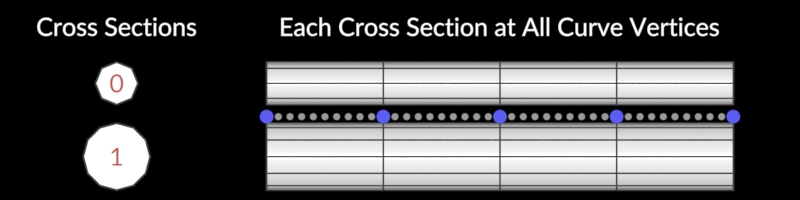Cycle Through Cross Section Primitives per Vertex

Within each single curve’s output grid, the cross section selected for each vertex will cycle through the cross sections, starting from the first cross section.

This is useful if the cross sections form all cross sections to be used along the curves in order, for example, if the cross section input contains all cross sections for a corridor in order.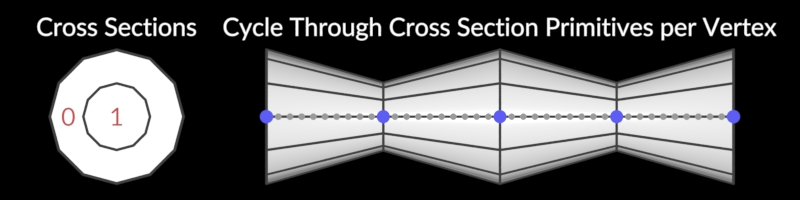Cycle Through Cross Section Primitives per Primitive

For each curve’s output grid, a single cross section will be selected, selecting the next cross section for each next curve.

This is useful if each curve has a different cross section to be used for it.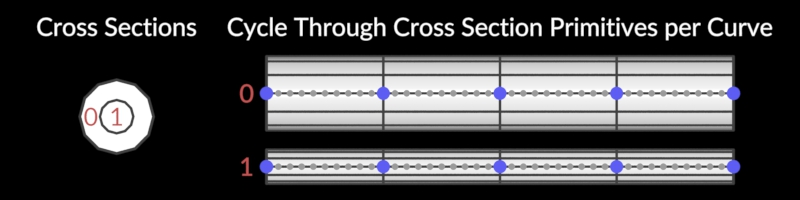Choose Cross Section Primitives by Attribute

For each vertex, select a cross section to use based on the attribute specified in the Cross Section Attribute parameter.Cross Section Attribute

When Cross Section Order is "Choose Cross Section Primitives by Attribute", this is the name of the attribute to use for selecting cross sections.

This can be a point, vertex, primitive, or detail attribute on the spine curve, or a primitive attribute on the cross-sections.

• If this is an integer attribute on the spine, it will select cross sections by primitive number.

• If this is an integer or string attribute present on both inputs, it will select a cross section with a matching value.

Primitive Type

The type of primitive to output (when Surface Type is not "Points"). "Automatic" generates the same type of surface as the input curves (for example, polylines create a polygon surface, NURBS curves produce a NURBS surface).

Ensure Unique Seam Vertices

If Surface Type is "Rows", "Columns", or "Rows and Columns", and the spine curve or cross-section is closed, this adds an extra vertex (if needed) to each curve to ensure that there are separate vertices for parametric curve u or v coordinate values 0 and 1.

If any output primitives are polygon soups, NURBS surfaces, Bezier surfaces, or bilinear mesh primitives, this option similarly also adds any extra vertices that would be needed to ensure that all uv seams are paramerized correctly.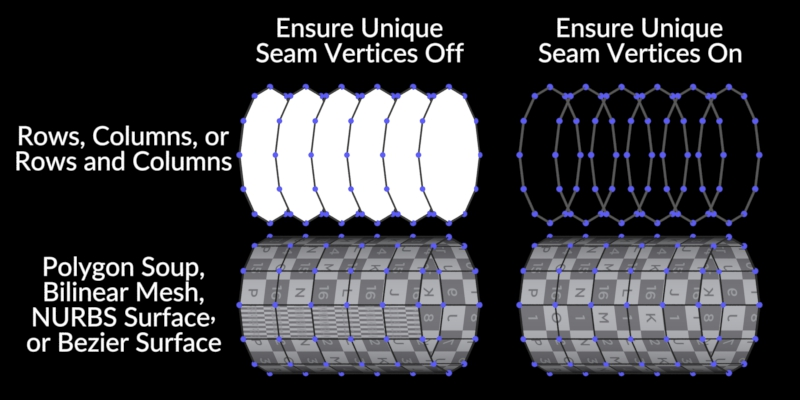Swap Rows and Columns

Adjusts the order of the points and primitives in the generated surface(s) to be column-major, having all points/primitives of one column before those of the next column, instead of the default (row-major, having all point/primitives of one row before those of the next row). Changing this also reverses normals, so you may also want to turn on Reverse Cross Sections to keep the current normals.

Close Implicit Backbone Curve if No Curve Input

If the node finds no spine curve in the first input, the node sweeps the cross sections in the second input as if there is a single curve with one vertex per cross section and all positions at the origin. When this is on, the node makes that implicit curve closed, treating the cross sections as forming a loop, instead of a line.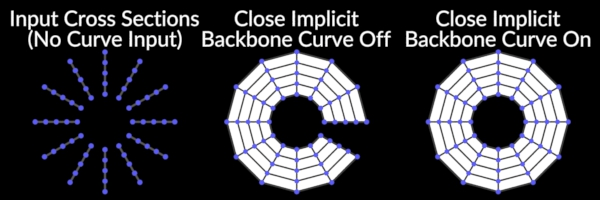Up Vectors

Target Up Vector

The initial orientation of the cross-section around the direction of the curve. You can use the roll, yaw, and pitch parameters on the Surface tab to further adjust the orientation of the cross-sections on top of this.

Curve Normal

Match the cross-section up-vector to the curve normal. This is useful when the curve is planar, it orients the cross-sections perpendicular to the plane.

X/Y/Z Axis

Match the cross-section up-vector to the X, Y, or Z axis.

Attribute

Get the up-vector from a per-curve value in a primitive attribute, or a shared value in a detail attribute. Set the attribute name in the Start up attribute parameter.

Custom

Match the cross-section up-vector to a custom vector. Set the custom vector in the Start up-vector parameter.

Target Up Vector at Start (else Average)

When this is on, at each point the node tries to get closest to the initial up-vector, while staying perpendicular to the spine curve tangent. When this is off, it tries to get closest to the average of all vertex up-vectors, instead of the initial up-vector.

Use Target End Up Vector

When Target up-vector at Start is on, and this is on, the node adjusts any twist along the spine to ensure the final up-vector is as close as possible to the target up-vector, without affecting the initial up-vector.

If Target up-vector is "Attribute" or "Custom", there are separate End Up Attribute and End Up Vector parameters, in order to specify different end up-vectors from the start up-vectors.

Start Up Attribute

When Target Up Vector is Attribute, this is the name of the primitive or detail attribute to use for each curve’s target up-vector.

End Up Attribute

When Target Up Vector is Attribute and Use Target End Up Vector is on, this is the name of the primitive or detail attribute that provides the target up-vectors at the end of the curve.

Start Up Vector

When Target up-vector is "Custom", this is the target up-vector at the end of the curve.

End up-vector

When Target up-vector is "Custom" and Use Target End Up Vector is on, this is the target up-vector at the end of the curve.

Tangents

Tangent Type

How to compute the spine curve’s tangent direction at each point. If Transform using curve point attributes is on and the spine curve has the required attributes, this is ignored.

(The Stretch Around Turns option always computes the stretch and stretch direction based on the "Average of Edge Directions" tangent, regardless of this setting.)

Average of Edge Directions

Average of the normalized directions of the previous and next edge.

Central Difference

Average of the non-normalized previous and next edge vectors.

This is similar to the "Central Difference" option for Velocity Approximation on theTrail node.

Previous Edge

Previous edge direction.

Next Edge

Next edge direction.

Z Axis (Ignore Curve)

The tangent will be chosen as the Z axis, regardless of the curve.

This can be useful if the cross sections have already been rotated to the desired orientation.Make Closed Curve Orientations Continuous

Any extra rotation required to make the start and end orientations match (either because the curve is non-planar, or you applied a total amount of twist that wasn’t an even multiple of 360) is spread out along the entire curve, instead of possibly coming suddenly along the last edge.

Extrapolate End Tangents

Extrapolate the tangent for the last edge from the previous two edges. When this is off, the tangent for the final edge is just the edge direction.

This is most useful when a backbone curve is intended to represent a circular arc, so the end cross sections will be rotated as expected.Transform Using Curve Point Attributes

Orient the cross-sections using the point attributes `P`, `N`, `up`, `orient`, `rot`, `trans`, `pivot`, `pscale`, `scale`, and/or `transform` on the spine curve.

• If `N` is present and none of `up`, `orient`, `rot`, or `transform` are present, the node uses `N` as the curve tangent and computes the rest of the rotation as usual.

• If `up` is present and none of `N`, `orient`, `rot`, or `transform` are present, the node computes the tangent as usual, and uses `up` as the up-vector at each vertex (before any roll or twist is applied).

## UVs and Attributes

UV Coordinates

Compute UVs

Generate a `uv` vertex attribute on the generated surface. If the input curves already have `uv` attributes, the node uses the values from those attributes (U corresponds to the cross sections, and V corresponds to the spine curve).

(If Surface Type is "Points", this will generate a point attribute.)

Override Any Existing UVs

When Compute UVs is on, compute new UVs for the surface, even if the input curves already had `uv` attributes.

Length-Weighted UVs

Scale the U and V coordinates based on proportional edge lengths so they're even across the whole surface, rather than bunching up where edges bunch up.

(If Normalize Computed Us and/or Normalize Computed Vs are on, the coordinates are still normalized to be within the 0 to 1 range.)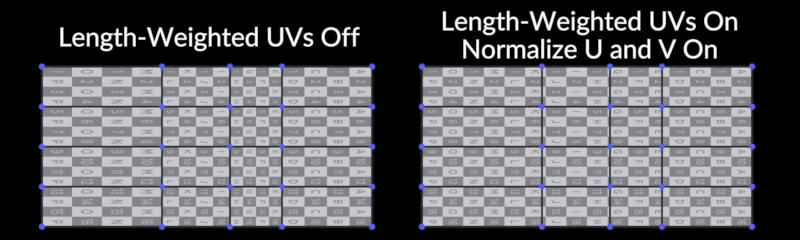Normalize Computed Us

Normalize computed U coordinates to be in the range 0 to 1 (before applying UV scale). When this is off, U corresponds to the average distance around the cross sections on the mesh (ignoring any stretch applied by Stretch Around Turns).

When this is on and Normalize Computed Vs is off, the node scales V such that U and V appear scaled similarly in space (depending on the Use Max Cross Section Length per Curve for Proportional Scale setting).

This is especially useful for things like applying repeating rope textures, where the texture should be applied isotropically.

Normalize Computed Vs

Normalize computed V coordinates to be in the range 0 to 1 (before applying UV scale). When this is off, V corresponds to the average distance along the surface in V, or the distance along the spine curve, depending on the Use Mesh Edge Lengths instead of Curve Edge Lengths parameter.

When this is on and Normalize Computed Us is off, the node scales U such that U and V appear scaled similarly in space.

Flip Computed Us

Reverse the direction of the computed U values (same as replacing each U value with `max(U values) - U`). Use this if a texture appears backward when applied to the surface.

This should be on for textures to appear correct on surfaces viewed from the front, (normals pointing toward the camera), and should be off for textures to appear correct on surfaces viewed from the back, (normals pointing away from the camera).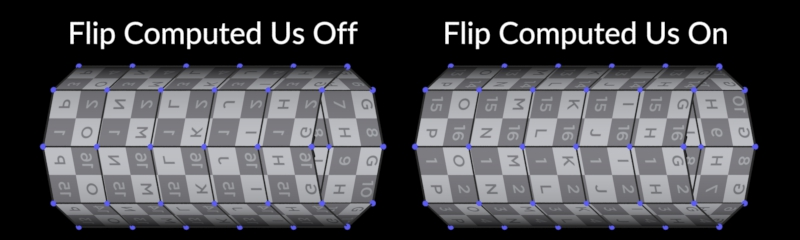UV Scale

UV Scale

A scale on the generated UV coordinates. This is applied after any normalization.

This is applied before the adjustments made by Make Computed Us Wrap Seamlessly and/or Make Computed Vs Wrap Seamlessly, so the texture will still wrap correctly.

Use Mesh Edge Lengths Instead of Curve Edge Lengths

When this is on and Length-Weighted UVs is on, the averages of the output mesh edge lengths in the curve direction are used as the lengths in the V direction, instead of the original curve edge lengths.

• This is useful when, for example, a curve has coincident points with different cross sections, such as a door jamb in a hallway, so that the surface between the two cross sections gets a non-zero area of texture space.

• It is also useful for generating UVs for grid end caps. However, it can be less stable, when changing rotations, than using the original curve lengths.Use Max Cross Section Length per Curve for Proportional Scale

When Normalize Computed Us is on and Normalize Computed Vs is on, this controls whether the node uses the maximum cross section length for computing the V scale, instead of the average of the previous and next cross section lengths for each V edge.

If the cross section length varies significantly, this can make the texture appear stretched in some places, but can also avoid instability and inconsistency in the V scale along the curve.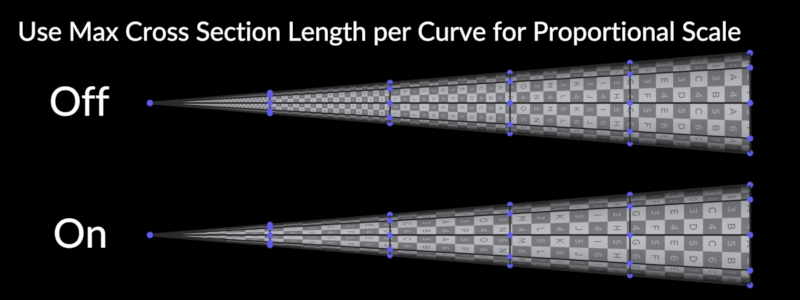UV Seams

Snap U to Nearest Boundary

When Normalize Computed Us is off and this is on, the node rounds the range of U to the nearest positive integer, so textures wrap correctly in the U direction. This node applies this rounding after the UV Scale.

Snap V to Nearest Boundary

When Normalize Computed Vs is off and this is on, the node roounds the range of V to the nearest positive integer, so textures wrap correctly in the V direction. This node applies this rounding after the UV Scale.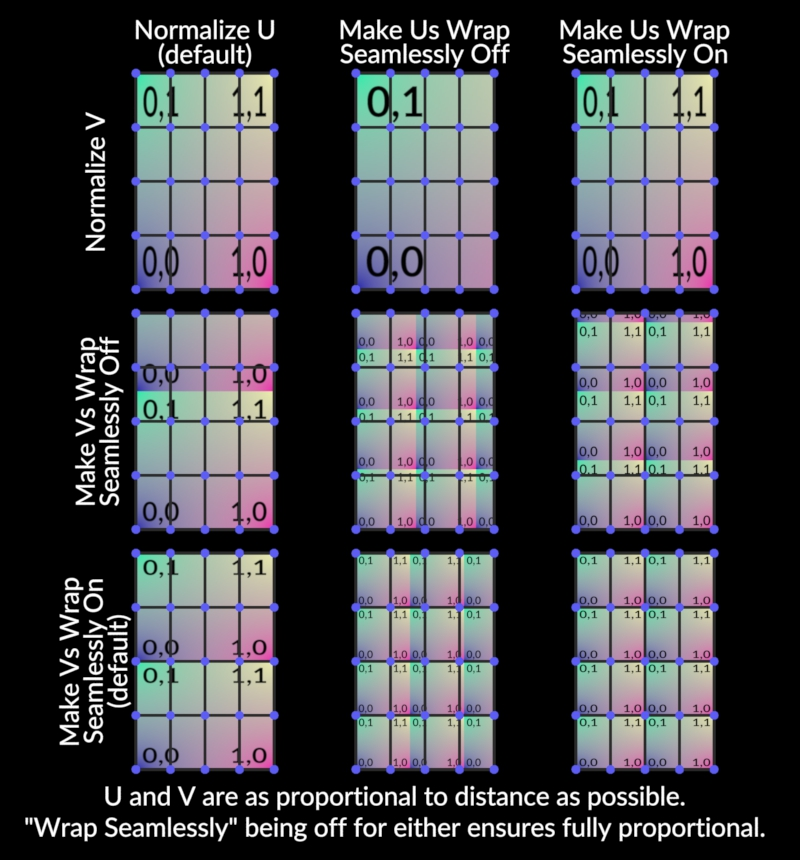Attributes

From Backbone Curves

Space-separated list of attributes to copy from the backbone curve onto the generated surface. You can use patterns here.

By default, `P`, `N`, `up`, `pscale`, `scale`, `orient`, `rot`, `pivot`, `trans`, and `transform` are not copied, to avoid copying attributes used for computing transforms.

From Cross Sections

Space-separated list of attributes to copy from the cross-section curves onto the generated surface. You can use patterns here.

The transforms attributes that have a transform type (position, vector, normal, quaternion, or transform matrix) appropriately.

Output attributesPoint Row Attribute

Create an integer point attribute with this name on the generated surface, containing the row number.

Point Col Attribute

Create an integer point attribute with this name on the generated surface, containing the column number.

Prim Row Attribute

Create an integer point attribute with this name on the generated surface, containing the quad row number.

Prim Col Attribute

Create an integer point attribute with this name on the generated surface, containing the quad column number.

Cross Section Num Attribute

Create an integer point attribute with this name on the generated surface, containing the primitive number of the cross section curve used to create the point.

Curve Num Attribute

Create an integer point attribute with this name on the generated surface, containing the primitive number of the spine curve used to create the point.

## Examples

SweepBasic Example for Sweep geometry node

This example demonstrates how the Sweep SOP copies geometry onto the points of a curve.

The Sweep SOP is unique in that it automatically places the copied geometry perpendicular to the backbone it is copied to. Variations such as the Cross Section’s scale can be adjusted using expressions.

SweepCurve Example for Sweep geometry node

This network contains an example of the Sweep SOP. A NURBS curve and NURBS circles are used as the backbone and the cross section geometries of the sweep operation respectively.

By controling the scaling of the cross section geometry in the Sweep SOP various effects can be acheived. Finally, a Skin SOP completes the form by using the swept geometry as a kind of skeleton.

SweepDome Example for Sweep geometry node

In this example the Sweep SOP a grid is used as the backbone of a sweep operation with arcs (created by a Circle SOP) as the hull (cross sections) of the sweep object.

The final sweep object is then skinned with a Skin SOP to create the dome geometry.

WigglyWorm Example for Sweep geometry node

This network demonstrates how the Sweep SOP can be used to construct geometry that is easily deformable. The Sweep SOP requires a backbone and cross section geometry.

Through a sin() function an expression is created to animate the backbone for a slithering effect. Then the circles are copied at every point on the backbone to create the skeleton of the worm. Finally, a simple skin operation completes the worm body.

# Geometry nodes

• Removes elements while trying to maintain the overall appearance.

• Creates Points or Polygons, or adds points/polys to an input.

• Creates agent primitives.

• Adds new clips to agent primitives.

• Defines how agents' animation clips should be played back.

• Creates geometry describing possible transitions between animation clips.

• Creates a new agent layer that is suitable for collision detection.

• Creates point attributes that specify the rotation limits of an agent’s joints.

• Builds a constraint network to hold an agent’s limbs together.

• Writes agent definition files to disk.

• Edits properties of agent primitives.

• Adds a new layer to agent primitives.

• Adjusts the head of an agent to look at a specific object or position.

• Adds various common point attributes to agents for use by other crowd nodes.

• Provides simple proxy geometry for an agent.

• Creates parent-child relationships between agents.

• Adapts agents' legs to conform to terrain and prevent the feet from sliding.

• Adds new transform groups to agent primitives.

• Extracts geometry from agent primitives.

• Extracts geometry from agent primitives for a Vellum simulation.

• Loads the geometry from an Alembic scene archive (.abc) file into a geometry network.

• Creates a geometry group for Alembic primitives.

• Modifies intrinsic properties of Alembic primitives.

• Aligns a group of primitives to each other or to an auxiliary input.

• Cleans up a series of break operations and creates the resulting pieces.

• Blurs out (or "relaxes") points in a mesh or a point cloud.

• Changes the size/precision Houdini uses to store an attribute.

• Composites vertex, point, primitive, and/or detail attributes between two or more selections.

• Copies attributes between groups of vertices, points, or primitives.

• Adds or edits user defined attributes.

• Deletes point and primitive attributes.

• Allows simple VEX expressions to modify attributes.

• Fades a point attribute in and out over time.

• Interpolates attributes within primitives or based on explicit weights.

• Copies and flips attributes from one side of a plane to another.

• Adds noise to attributes of the incoming geometry.

• Interactively paint point attributes, such as color or deformation mask values, directly on geometry.

• Promotes or demotes attributes from one geometry level to another.

• Generates random attribute values of various distributions.

• Fits an attribute’s values to a new range.

• Renames or deletes point and primitive attributes.

• Modifies point attributes based on differences between two models.

• Edits string attribute values.

• Copies, moves, or swaps the contents of attributes.

• Transfers vertex, point, primitive, and/or detail attributes between two models.

• Transfers attributes between two geometries based on UV proximity.

• Runs a VOP network to modify geometry attributes.

• Runs a VEX snippet to modify attribute values.

• Samples texture map information to a point attribute.

• Copies information from a volume onto the point attributes of another piece of geometry, with optional remapping.

• Converts primitives for ODE and Bullet solvers.

• Computes lighting values within volume primitives

• Provides operations for moving knots within the parametric space of a NURBS curve or surface.

• Applies captures such as bend, twist, taper, and squash/stretch.

• Deletes primitives, points, edges or breakpoints.

• Computes a 3D metamorphosis between shapes with the same topology.

• The start of a looping block.

• The start of a compile block.

• The end/output of a looping block.

• The end/output of a compile block.

• Supports Bone Deform by assigning capture weights to bones.

• Supports Bone Deform by assigning capture weights to points based on biharmonic functions on tetrahedral meshes.

• Utility node that supports Bone Capture Biharmonic by creating lines from bones with suitable attributes.

• Supports Bone Deform by assigning capture weights to points based on distance to bones.

• Uses capture attributes created from bones to deform geometry according to their movement.

• Creates default geometry for Bone objects.

• Combines two polygonal objects with boolean operators, or finds the intersection lines between two polygonal objects.

• Fractures the input geometry using cutting surfaces.

• Creates a bounding box, sphere, or rectangle for the input geometry.

• Creates a cube or six-sided rectangular box.

• Deforms the points in the first input using one or more magnets from the second input.

• Imports 2d geometry from a Composite network.

• Records and caches its input geometry for faster playback.

• Closes open areas with flat or rounded coverings.

• Converts array attributes into a single index-pair capture attribute.

• Converts a single index-pair capture attribute into per-point and detail array attributes.

• Adjusts capture regions and capture weights.

• Lets you paint capture attributes directly onto geometry.

• Copies capture attributes from one half of a symmetric model to the other.

• Overrides the capture weights on individual points.

• Supports Capture and Deform operation by creating a volume within which points are captured to a bone.

• Slices, cuts or extracts points or cross-sections from a primitive.

• Reads sample data from a chop and converts it into point positions and point attributes.

• Creates open or closed arcs, circles and ellipses.

• Transforms selected geometry into a circle.

• Lets you deform NURBS faces and NURBS surfaces by pulling points that lie directly on them.

• Helps clean up dirty models.

• Removes or groups geometry on one side of a plane, or creases geometry along a plane.

• Captures low-res simulated cloth.

• Deforms geometry captured by the Cloth Capture SOP.

• Creates a volume representation of source geometry.

• Fills a volume with a diffuse light.

• Applies a cloud like noise to a Fog volume.

• Low-level machinery to cluster points based on their positions (or any vector attribute).

• Higher-level node to cluster points based on their positions (or any vector attribute).

• Creates geometry and VDB volumes for use with DOPs collisions.

• Adds color attributes to geometry.

• Adjust surface point normals by painting.

• Creates lines between nearby pieces.

• Creates an attribute with a unique value for each set of connected primitives or points.

• Creates simple geometry for use as control shapes.

• Converts geometry from one geometry type to another.

• Converts a 2D height field to a 3D VDB volume, polygon surface, or polygon soup surface.

• Converts the input geometry into line segments.

• Polygonizes metaball geometry.

• Generates the oriented surface of a tetrahedron mesh.

• Converts sparse volumes.

• Converts a Point Cloud into a VDB Points Primitive, or vice versa.

• Converts the iso-surface of a volume into a polygonal surface.

• Decomposes the input geometry into approximate convex segments.

• Creates multiple copies of the input geometry, or copies the geometry onto the points of the second input.

• Copies geometry and applies transformations to the copies.

• Copies geometry in the first input onto curves of the second input.

• Copies geometry in the first input onto the points of the second input.

• Manually adds or removes a creaseweight attribute to/from polygon edges, for use with the Subdivide SOP.

• Deforms and animates a piece of geometry across a surface.

• Creates a surface around cross sections.

• Populates a crowd of agent primitives.

• Creates polygonal, NURBS, or Bezier curves.

• Deforms a spline surface by reshaping a curve on the surface.

• Finds the intersections (or points of minimum distance) between two or more curves or faces.

• Imports fields from DOP simulations, saves them to disk, and loads them back again.

• Imports scalar and vector fields from a DOP simulation.

• Imports option and record data from DOP simulations into points with point attributes.

• Generates point emission sources for debris from separating fractured rigid body objects.

• Runs a VEX snippet to deform geometry.

• Deletes input geometry by group, entity number, bounding volume, primitive/point/edge normals, and/or degeneracy.

• Smooths out (or "relaxes") point deformations.

• Attempts to prevent collisions when deforming geometry.

• Deletes edges from the input polygonal geometry merging polygons with shared edges.

• Measures the distance of the shortest path along the geometry’s edges or surfaces from each start point.

• Measures distance between each point and a reference geometry.

• Measures distance of each point from a target.

• Divides, smooths, and triangulates polygons.

• Imports and transforms geometry based on information extracted from a DOP simulation.

• Creates a curve based on user input in the viewport.

• Culls the input geometry according to the specifications of the For Each SOP.

• Collapses edges and faces to their centerpoints.

• Sharpens edges by uniquing their points and recomputing point normals.

• Inserts points on the edges of polygons and optionally connects them.

• Transforms selected edges so that all edges are of equal length.

• Flips the direction of polygon edges.

• Cuts geometry along edges using guiding curves.

• Straightens selected edges.

• Copies and optionally modifies attribute values along edges networks and curves.

• Edits points, edges, or faces interactively.

• Closes, opens, or clamps end points.

• Sets an attribute on selected points or primitives to sequential numbers or strings.

• Generates a message, warning, or error, which can show up on a parent asset.

• Pushes geometry out from the center to create an exploded view.

• Export transform attributes to object nodes.

• Computes the centroid of each piece of the geometry.

• Computes the best-fit transform between two pieces of geometry.

• Extrudes geometry along a normal.

• Extrudes surface geometry into a volume.

• Creates a surface or density VDB for sourcing FLIP simulations.

• Controls the smoothness of faceting of a surface.

• Evolves polygonal curves as vortex filaments.

• Reads, writes, or caches geometry on disk.

• Writes and reads geometry sequences to disk.

• Reads and collates data from disk.

• Creates smooth bridging geometry between two curves or surfaces.

• Finds the shortest paths from start points to end points, following the edges of a surface.

• Fits a spline curve to points, or a spline surface to a mesh of points.

• Compresses the output of fluid simulations to decrease size on disk

• Creates 3D text from Type 1, TrueType and OpenType fonts.

• Uses a metaball to attract or repel points or springs.

• Creates jagged mountain-like divisions of the input geometry.

• Creates a set of hair-like curves across a surface.

• Merges points.

• Geometry nodes live inside Geo objects and generate geometry.

• Adds strength to a glue constraint network according to cluster values.

• Generates particles to be used as sources in a particle-based grain simulation.

• Assigns a unique integer attribute to non-touching components.

• Creates planar geometry.

• Blends the guides and skin of two grooms.

• Fetches groom data from grooming objects.

• Packs the components of a groom into a set of named Packed Primitives for the purpose of writing it to disk.

• Switches between all components of two groom streams.

• Unpacks the components of a groom from a packed groom.

• Generates groups of points, primitives, edges, or vertices according to various criteria.

• Combines point groups, primitive groups, or edge groups according to boolean operations.

• Copies groups between two pieces of geometry, based on point/primitive numbers.

• Deletes groups of points, primitives, edges, or vertices according to patterns.

• Expands or shrinks groups of Edges, Points, Primitives, or Vertices.

• Runs VEX expressions to modify group membership.

• Constructs groups for paths between elements.

• Sets group membership interactively by painting.

• Converts point, primitive, edge, or vertex groups into point, primitive, edge, or vertex groups.

• Groups points and primitives by ranges.

• Renames groups according to patterns.

• Transfers groups between two pieces of geometry, based on proximity.

• Groups points and primitives by lasso.

• Creates a group that includes the boundaries of the specified attribute.

• Advects guide points through a velocity volume.

• Resolves collisions of guide curves with VDB signed distance fields.

• Deforms geometry with an animated skin and optionally guide curves.

• Allows intuitive manipulation of guide curves in the viewport.

• Creates standard primitive groups used by grooming tools.

• Quickly give hair guides some initial direction.

• Creates masking attributes for other grooming operations.

• Creates and prepares parting lines for use with hair generation.

• Looks up skin geometry attributes under the root point of guide curves.

• Constructs a coherent tangent space along a curve.

• Transfer hair guides between geometries.

• Converts dense hair curves to a polygon card, keeping the style and shape of the groom.

• Clumps guide curves together.

• Generates hair on a surface or from points.

• Generates a velocity field based on stroke primitives.

• Generates an initial heightfield volume for use with terrain tools.

• Blurs a terrain height field or mask.

• Limits height values to a certain minimum and/or maximum.

• Creates a copy of a height field or mask.

• Extracts a square of a certain width/length from a larger height volume, or resizes/moves the boundaries of the height field.

• Creates a cutout on a terrain based on geometry.

• Displaces a height field by another field.

• Advects the input volume through a noise pattern to break up hard edges and add variety.

• Lets you draw shapes to create a mask for height field tools.

• Calculates thermal and hydraulic erosion over time (frames) to create more realistic terrain.

• Simulates the erosion from one heightfield sliding over another for a short time.

• Distributes water along a heightfield. Offers controls for adjusting the intensity, variability, and location of rainfall.

• Calculates the effect of thermal erosion on terrain for a short time.

• Imports a 2D image map from a file or compositing node into a height field or mask.

• Generates flow and flow direction layers according to the input height layer.

• Copies another layer over the mask layer, and optionally flattens the height field.

• Composites together two height fields.

• Sets all values in a heightfield layer to a fixed value.

• Sets the border voxel policy on a height field volume.

• Creates a mask based on different features of the height layer.

• Creates a mask based some other geometry.

• Creates a mask where the input terrain is hollow/depressed, for example riverbeds and valleys.

• Adds vertical noise to a height field, creating peaks and valleys.

• Exports height and/or mask layers to disk as an image.

• Lets you paint values into a height or mask field using strokes.

• Patches features from one heightfield to another.

• Adds displacement in the form of a ramps, steps, stripes, Voronoi cells, or other patterns.

• Projects 3D geometry into a height field.

• Applies a material that lets you plug in textures for different layers.

• Remaps the values in a height field or mask layer.

• Changes the resolution of a height field.

• Scatters points across the surface of a height field.

• Simulates loose material sliding down inclines and piling at the bottom.

• Creates stepped plains from slopes in the terrain.

• Stitches height field tiles back together.

• Splits a height field volume into rows and columns.

• Height field specific scales and offsets.

• Visualizes elevations using a custom ramp material, and mask layers using tint colors.

• Makes holes in surfaces.

• Deforms the points in the first input to make room for the inflation tool.

• Instances Geometry on Points.

• Creates points with attributes at intersections between a triangle and/or curve mesh with itself, or with an optional second set of triangles and/or curves.

• Composes triangle surfaces and curves together into a single connected mesh.

• Processes its inputs using the operation of a referenced compiled block.

• Builds an offset surface from geometry.

• Generates an isometric surface from an implicit function.

• The Join op connects a sequence of faces or surfaces into a single primitive that inherits their attributes.

• Divides, deletes, or groups geometry based on an interactively drawn line.

• Creates fractal geometry from the recursive application of simple rules.

• Deforms geometry based on how you reshape control geometry.

• Reads a lidar file and imports a point cloud from its data.

• Creates polygon or NURBS lines from a position, direction, and distance.

• Animates points using an MDD file.

• Deforms geometry by using another piece of geometry to attract or repel points.

• Aligns the input geometry to a specific axis.

• Resizes and recenters the geometry according to reference geometry.

• Reorders the primitive and point numbers of the input geometry to match some reference geometry.

• Assigns one or more materials to geometry.

• Measures area, volume, or curvature of individual elements or larger pieces of a geometry and puts the results in attributes.

• Merges geometry from its inputs.

• Defines groupings of metaballs so that separate groupings are treated as separate surfaces when merged.

• Creates metaballs and meta-superquadric surfaces.

• Duplicates and mirrors geometry across a mirror plane.

• Displaces points along their normals based on fractal noise.

• Supports Muscle Deform by assigning capture weights to points based on distance away from given primitives

• Deforms a surface mesh representing skin to envelop or drape over geometry representing muscles

• Creates a "naming" attribute on points or primitives allowing you to refer to them easily, similar to groups.

• Computes surface normal attribute.

• Does nothing.

• Merges geometry from multiple sources and allows you to define the manner in which they are grouped together and transformed.

• Assists the creation of a Muscle or Muscle Rig by allowing you to draw a stroke on a projection surface.

• Deforms input geometry based on ocean "spectrum" volumes.

• Generates particle-based foam

• Generates particles and volumes from ocean "spectrum" volumes for use in simulations

• Generates volumes containing information for simulating ocean waves.

• Instances individual waveforms onto input points and generated points.

• Executes an OpenCL kernel on geometry.

• Computes orientations (frames) along curves.

• Marks the output of a sub-network.

• Packs geometry into an embedded primitive.

• Packs points into a tiled grid of packed primitives.

• Editing Packed Disk Primitives.

• Editing Packed Primitives.

• Creates a color volume based on drawn curve

• Creates a fog volume based on drawn curve

• Creates an SDF volume based on drawn curve

• Generates a surface around the particles from a particle fluid simulation.

• Creates a set of regular points filling a tank.

• Places points and primitives into groups based on a user-supplied rule.

• Deforms geometry using the shape of a curve.

• Moves primitives, points, edges or breakpoints along their normals.

• Creates a planar polygonal patch.

• Fills in a 2d curve network with triangles.

• Deforms flat geometry into a pleat.

• Creates platonic solids of different types.

• Constructs an iso surface from its input points.

• Deforms geometry on an arbitrary connected point mesh.

• Creates new points, optionally based on point positions in the input geometry.

• Jitters points in random directions.

• Moves points with overlapping radii away from each other, optionally on a surface.

• Generates a cloud of points around the input points.

• Splits points shared by multiple vertices, optionally only if the vertices differ in attribute values.

• Computes and manipulates velocities for points of a geometry.

• Merges points interactively.

• Creates set of regular points filling a volume.

• Creates flat or tube-shaped polygon surfaces between source and destination edge loops, with controls for the shape of the bridge.

• Creates offset polygonal geometry for planar polygonal graphs.

• Extrudes polygonal faces and edges.

• Creates straight, rounded, or custom fillets along edges and corners.

• Breaks curves where an attribute crosses a threshold.

• Helps repair invalid polygonal geometry, such as for cloth simulation.

• Fills holes with polygonal patches.

• Creates coordinate frame attributes for points and vertices.

• Creates new polygons using existing points.

• Creates a smooth polygonal patch from primitives.

• Cleans up topology of polygon curves.

• Reduces the number of polygons in a model while retaining its shape. This node preserves features, attributes, textures, and quads during reduction.

• Combines polygons into a single primitive that can be more efficient for many polygons

• The PolySpline SOP fits a spline curve to a polygon or hull and outputs a polygonal approximation of that spline.

• Divides an existing polygon into multiple new polygons.

• Constructs polygonal tubes around polylines, creating renderable geometry with smooth bends and intersections.

• Interpolates between a set of pose-shapes based on the value of a set of drivers.

• Combine result of Pose-Space Deform with rest geometry.

• Packs geometry edits for pose-space deformation.

• Creates common attributes used by the Pose-Space Edit SOP.

• Edits primitive, primitive attributes, and profile curves.

• Extracts or manipulates profile curves.

• Creates profile curves on surfaces.

• Applies common post-processing effects to the results of Pyro solves.

• Runs a dynamic Pyro simulation.

• Creates points for sourcing pyro and smoke simulations.

• Pyro Source Spread solves for the spreading of flame across a point cloud.

• Runs a Python snippet to modify the incoming geometry.

• Runs a dynamic Bullet simulation.

• Combines fractured pieces or constraints into larger clusters.

• Packs and creates attributes describing rigid body objects.

• Stores the primitive number and distance to the opposite face on the inside faces of fractured geometry.

• Creates attributes describing rigid body constraints.

• Creates rigid body constraint geometry from curves drawn in the viewport.

• Creates rigid body constraint geometry from interactively drawn lines in the viewport.

• Creates rigid body constraint geometry from a set of rules and conditions.

• Converts existing constraint prims into constraints with different anchor positions.

• Deforms geometry with simulated proxy geometry.

• Detects when connected faces have become separated.

• Merges RBD fractured geometry with the proxy geometry and pushes it out from the center to create an exploded view.

• Packs RBD fractured geometry, saves them to disk, and loads them back again.

• Creates additional detail on the interior surfaces of fractured geometry.

• Fractures the input geometry based on a material type.

• Packs RBD geometry, constraints, and proxy geometry into a single geometry.

• Paints values onto geometry or constraints using strokes.

• Unpacks an RBD setup into three outputs.

• Attaches RenderMan shaders to groups of faces.

• Generates surfaces by stretching cross-sections between two guide rails.

• Projects one surface onto another.

• Increases the number of points/CVs in a curve or surface without changing its shape.

• Scatters new guides, interpolating the properties of existing guides.

• Recreates the shape of the input surface using "high-quality" (nearly equilateral) triangles.

• Repacks geometry as an embedded primitive.

• Resamples one or more curves or surfaces into even length segments.

• Sets the alignment of solid textures to the geometry so the texture stays put on the surface as it deforms.

• Retimes the time-dependent input geometry.

• Reverses or cycles the vertex order of faces.

• Revolves a curve around a center axis to sweep out a surface.

• Rewires vertices to different points specified by an attribute.

• Generates ripples by displacing points along the up direction specified.

• Generates ripples by displacing points along the up direction specified.

• Scatters new points randomly across a surface or through a volume.

• Runs scripts when cooked.

• Lets you interactively reshape a surface by brushing.

• Morphs though a sequence of 3D shapes, interpolating geometry and attributes.

• Computes the post-deform or pre-deform difference of two geometries with similar topologies.

• Computes the convex hull of the input geometry and moves its polygons inwards along their normals.

• Builds a skin surface between any number of shape curves.

• Creates a sky filled with volumentric clouds

• Smooths out (or "relaxes") polygons, meshes and curves without increasing the number of points.

• Moves the selected point along its normal, with smooth rolloff to surrounding points.

• Moves the selected point, with smooth rolloff to surrounding points.

• Creates a tetrahedral mesh that conforms to a connected mesh as much as possible.

• Creates a simple tetrahedral mesh that covers a connected mesh.

• Creates a partition of a tetrahedral mesh that can be used for finite-element fracturing.

• Allows running a SOP network iteratively over some input geometry, with the output of the network from the previous frame serving as the input for the network at the current frame.

• Reorders points and primitives in different ways, including randomly.

• Creates a sphere or ovoid surface.

• Splits primitives or points into two streams.

• Spray paints random points onto a surface.

• A SOP node that sets the sprite display for points.

• Insets points on polygonal faces.

• Caches the input geometry in the node on command, and then uses it as the node’s output.

• Stretches two curves or surfaces to cover a smooth area.

• Low level tool for building interactive assets.

• Subdivides polygons into smoother, higher-resolution polygons.

• The Subnet op is essentially a way of creating a macro to represent a collection of ops as a single op in the Network Editor.

• Trims or creates profile curves along the intersection lines between NURBS or bezier surfaces.

• Creates a surface by sweeping a cross section curve along a spine curve.

• Switches between network branches based on an expression or keyframe animation.

• Switches between two network branches based on an expression or geometry test.

• Sends input geometry to a TOP subnet and retrieves the output geometry.

• Reads a CSV file creating point per row.

• Creates a rock creature, which can be used as test geometry.

• Creates a pig head, which can be used as test geometry..

• Creates a rubber toy, which can be used as test geometry.

• Creates a shader ball, which can be used to test shaders.

• Creates a squab, which can be used as test geometry.

• Creates a template body, which can be used as test geometry.

• Creates a template head, which can be used as test geometry.

• Creates a soldier, which can be used as test geometry.

• Provides a simple crowd simulation for testing transitions between animation clips.

• Provides a simple Bullet simulation for testing the behavior of a ragdoll.

• Partitions a given tetrahedron mesh into groups of tets isolated by a given polygon mesh

• Performs variations of a Delaunay Tetrahedralization.

• Cooks the input at a different time.

• Sets attributes used by the Toon Color Shader and Toon Outline Shader.

• Non-rigidly deforms a surface to match the size and shape of a different surface.

• Lets you interactively draw a reduced quad mesh automatically snapped to existing geometry.

• Creates a torus (doughnut) shaped surface.

• Traces curves from an image file.

• Creates trails behind points.

• The Transform operation transforms the source geometry in "object space" using a transformation matrix.

• Transforms the input geometry relative to a specific axis.

• Transforms the input geometry by a point attribute.

• Transforms input geometry according to transformation attributes on template geometry.

• Creates a triangular Bezier surface.

• Refines triangular meshes using various metrics.

• Connects points to form well-shaped triangles.

• Trims away parts of a spline surface defined by a profile curve or untrims previous trims.

• Creates open or closed tubes, cones, or pyramids.

• Generates an edge group representing suggested seams for flattening a polygon model in UV space.

• Adjusts texture coordinates in the UV viewport by painting.

• Lets you interactively move UVs in the texture view.

• Creates flattened pieces in texture space from 3D geometry.

• Merges UVs.

• Packs UV islands efficiently into a limited area.

• Relaxes UVs by pulling them out toward the edges of the texture area.

• Assigns UVs by projecting them onto the surface from a set direction.

• Applies an image file as a textured shader to a surface.

• Assigns texture UV coordinates to geometry for use in texture and bump mapping.

• Transforms UV texture coordinates on the source geometry.

• Separates UVs into reasonably flat, non-overlapping groups.

• Processes geometry using an external program.

• Unpacks packed primitives.

• Unpacks points from packed primitives.

• Converts "packed USD" primitives into normal Houdini geometry.

• Creates one or more empty/uniform VDB volume primitives.

• Activates voxel regions of a VDB for further processing.

• Expand or contract signed distance fields stored on VDB volume primitives.

• Moves VDBs in the input geometry along a VDB velocity field.

• Moves points in the input geometry along a VDB velocity field.

• Computes an analytic property of a VDB volumes, such as gradient or curvature.

• Clips VDB volume primitives using a bounding box or another VDB as a mask.

• Combines the values of two aligned VDB volumes in various ways.

• Tests VDBs for Bad Values and Repairs.

• Cuts level set VDB volume primitives into multiple pieces.

• Build an LOD Pyramid from a VDB.

• Blends between source and target SDF VDBs.

• Create a mask of the voxels in shadow from a camera for VDB primitives.

• Deletes points inside of VDB Points primitives.

• Manipulates the Internal Groups of a VDB Points Primitive.

• Computes the steady-state air flow around VDB obstacles.

• Removes divergence from a Vector VDB.

• Fixes signed distance fields stored in VDB volume primitives.

• Re-samples a VDB volume primitive into a new orientation and/or voxel size.

• Reshapes signed distance fields in VDB volume primitives.

• Splits SDF VDBs into connected components.

• Smooths out the values in a VDB volume primitive.

• Smooths out SDF values in a VDB volume primitive.

• Creates an SDF VDB based on the active set of another VDB.

• Merges three scalar VDB into one vector VDB.

• Splits a vector VDB primitive into three scalar VDB primitives.

• Replaces a VDB volume with geometry that visualizes its structure.

• Generates a signed distance field (SDF) VDB volume representing the surface of a set of particles from a particle fluid simulation.

• Converts point clouds and/or point attributes into VDB volume primitives.

• Converts polygonal surfaces and/or surface attributes into VDB volume primitives.

• Fills a VDB volume with adaptively-sized spheres.

• Configures geometry for Vellum Grain constraints.

• Configure constraints on geometry for the Vellum solvers.

• Vellum solver setup to pre-roll fabric to drape over characters.

• Packs Vellum simulations, saves them to disk, and loads them back again.

• Packs Vellum geometry and constraints into a single geometry.

• Applies common post-processing effects to the result of Vellum solves.

• Ties vellum points to a reference frame defined by moving geometry.

• Blends the current rest values of constraints with a rest state calculated from external geometry.

• Runs a dynamic Vellum simulation.

• Unpacks a Vellum simulation into two outputs.

• Verify that a bsdf conforms to the required interface.

• Manually adds or edits attributes on vertices (rather than on points).

• Shows/hides primitives in the 3D viewer and UV editor.

• Lets you attach visualizations to different nodes in a geometry network.

• Creates a volume primitive.

• Computes analytic properties of volumes.

• Computes a speed-defined travel time from source points to voxels.

• Blurs the voxels of a volume.

• Bounds voxel data.

• Cuts polygonal objects using a signed distance field volume.

• Re-compresses Volume Primitives.

• Convolves a volume by a 3×3×3 kernel.

• Compute the Fast Fourier Transform of volumes.

• Feathers the edges of volumes.

• Flattens many volumes into one volume.

• Combines the scalar fields of volume primitives.

• Translates the motion between two "image" volumes into displacement vectors.

• Fill in a region of a volume with features from another volume.

• Remaps a volume according to a ramp.

• Rasterizes into a volume.

• Samples point attributes into VDBs.

• Converts a curve into a volume.

• Converts fur or hair to a volume for rendering.

• Converts a point cloud into a volume.

• Converts a point cloud into a volume.

• Reduces the values of a volume into a single number.

• Resamples the voxels of a volume to a new resolution.

• Resizes the bounds of a volume without changing voxels.

• Builds a Signed Distance Field from an isocontour of a volume.

• Extracts 2d slices from volumes.

• Splices overlapping volume primitives together.

• Stamps volumes instanced on points into a single target volume.

• Adaptively surfaces a volume hierarchy with a regular triangle mesh.

• Computes a trail of points through a velocity volume.

• Runs CVEX on a set of volume primitives.

• Computes a velocity volume.

• Generates a volume velocity field using curve tangents.

• Generates a velocity field within a surface geometry.

• Adjusts attributes for multi-volume visualization.

• Runs a VEX snippet to modify voxel values in a volume.

• Sets the voxels of a volume from point attributes.

• Fractures the input geometry by performing a Voronoi decomposition of space around the input cell points

• Given an object and points of impact on the object, this SOP generates a set of points that can be used as input to the Voronoi Fracture SOP to simulate fracturing the object from those impacts.

• Cuts the geometry into small pieces according to a set of cuts defined by polylines.

• Creates the point attributes needed to create a Vortex Force DOP.

• Generates volumes to be used as sources in a whitewater simulation.

• Computes generalized winding number of surface at query points.

• Morphs between curve shapes while maintaining curve length.

• Captures surfaces to a wire, allowing you to edit the wire to deform the surface.

• Deforms geometry captured to a curve via the Wire Capture node.

• Transfers the shape of one curve to another.

• Constructs polygonal tubes around polylines, creating renderable geometry.

• Assigns channel paths and/or pickscripts to geometry.